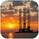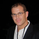จำนวนเข้าชม 3464
There is an important information the Squeeze indicator is missing, which is the Pre Squeeze entry. While the Bollinger band begins to curves out of the KC, The breakout usually happens. There are many instances that the Squeeze indicator will fire, after the Major move, I cant blame the indicator, thats the nature (lagging) of all indicators, and we have to live with it.

Therefore pre-squeeze-fire Entry can be critical in timing your entry. Timing it too early could result in stoploss if it turns against you, ( or serious burn on options premium), because we never know when the squeeze will fire with the TTM squeeze, But now We know. Its a little timing tool. Managing position is critical when playing options.

I will code the timing signal when I get some time.

Updated Versions -
study(title="UCS_Squeeze V1", shorttitle="UCS_SQZ-V1", overlay=false)
source = close
useTrueRange = input(true)
length = input(20, minval=1)
multkc = input(1.5)
ma = ema(source, length)
range = useTrueRange ? tr : high - low
rangema = ema(range, length)
upperkc = sma((0 + rangema * multkc),5)
lowerkc = sma((0 - rangema * multkc),5)
c = blue
u = plot(upperkc, color=c, title="Upper")
//plot(ma, color=c, title="Basis")
l = plot(lowerkc, color=c, title="Lower")
fill(u, l, color=c)

multbb = input(2.0, minval=0.001, maxval=50)
basis = sma(source, length)
dev = multbb * stdev(source, length)
upperbb = sma((0 + dev),5)
lowerbb = sma((0 - dev),5)
//plot(basis, color=red)
p1 = plot(upperbb, color=green)
p2 = plot(lowerbb, color=green)
fill(p1, p2, color = green)

sqzOn  = (lowerbb > lowerkc) and (upperbb < upperkc)
sqzOff = (lowerbb < lowerkc) and (upperbb > upperkc)
noSqz  = (sqzOn == false) and (sqzOff == false)

scolor = noSqz ? blue : sqzOn ? red : green
plot(0, color=scolor, style=circles, linewidth=3, title = "Squeeze Status")

## ความคิดเห็นFood for thoughts - No indicator can be perfect. This is an extreme example.
ตอบกลับAlgyros

Just to explain, I added Bollinger Band Width with a moving average signal. The idea is to take the trade as the volatility expands. Hope it helps.
ตอบกลับUSC - I just found you Squeeze V1 - looks great as well - thank again for all of your mentoring and guidance!!!
ตอบกลับ